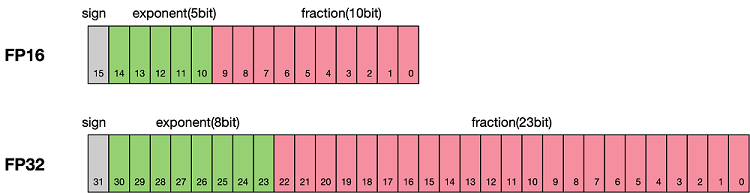# 混合精度¶

Ascend GPU 模型调优

## 浮点数据类型¶• 最高位表示符号位sign bit。

• 中间表示指数位exponent bit。

• 低位表示分数位fraction bit。

$x=(-1)^{S}\times2^{E-15}\times(1+\frac{fraction}{1024})$

$x=(-1)^{S}\times2^{E-127}\times(1.M)$

$x=(-1)^{S}\times2^{E-1023}\times(1.M)$

FP16可以表示的最大值为 0 11110 1111111111，计算方法为：

$(-1)^0\times2^{30-15}\times1.1111111111 = 1.1111111111(b)\times2^15 = 1.9990234375(d)\times2^15 = 65504$

FP16可以表示的最小值为 0 00001 0000000000，计算方法为：

$(-1)^{1}\times2^{1-15}=2^{-14}=6.104×10^{-5}=-65504$

## 使用FP16训练问题¶

• 减少内存占用：FP16的位宽是FP32的一半，因此权重等参数所占用的内存也是原来的一半，节省下来的内存可以放更大的网络模型或者使用更多的数据进行训练。

• 加快通讯效率：针对分布式训练，特别是在大模型训练的过程中，通讯的开销制约了网络模型训练的整体性能，通讯的位宽少了意味着可以提升通讯性能，减少等待时间，加快数据的流通。

• 计算效率更高：在特殊的AI加速芯片如华为昇腾系列的Ascend 910和310系列，或者NVIDIA VOLTA架构的GPU上，使用FP16的执行运算性能比FP32更加快。

• 数据溢出：数据溢出比较好理解，FP16的有效数据表示范围为 $$[6.10\times10^{-5}, 65504]$$，FP32的有效数据表示范围为 $$[1.4\times10^{-45}, 1.7\times10^{38}]$$。可见FP16相比FP32的有效范围要窄很多，使用FP16替换FP32会出现上溢（Overflow）和下溢（Underflow）的情况。而在深度学习中，需要计算网络模型中权重的梯度（一阶导数），因此梯度会比权重值更加小，往往容易出现下溢情况。

• 舍入误差：Rounding Error指示是当网络模型的反向梯度很小，一般FP32能够表示，但是转换到FP16会小于当前区间内的最小间隔，会导致数据溢出。如0.00006666666在FP32中能正常表示，转换到FP16后会表示成为0.000067，不满足FP16最小间隔的数会强制舍入。

## 混合精度计算流程¶

MindSpore混合精度典型的计算流程如下图所示：1. 参数以FP32存储；

2. 正向计算过程中，遇到FP16算子，需要把算子输入和参数从FP32 cast成FP16进行计算；

3. 将Loss层设置为FP32进行计算；

4. 反向计算过程中，首先乘以Loss Scale值，避免反向梯度过小而产生下溢；

5. FP16参数参与梯度计算，其结果将被cast回FP32；

6. 除以Loss scale值，还原被放大的梯度；

7. 判断梯度是否存在溢出，如果溢出则跳过更新，否则优化器以FP32对原始参数进行更新。

## MindSpore混合精度¶

### 自动混合精度¶

BatchNorm算子和Loss涉及到的算子外因为精度问题，仍然使用FP32执行运算。

1. 引入MindSpore的模型训练接口Model

2. 定义网络：该步骤和正常的网络定义相同(无需新增任何配置)；

3. 创建数据集：该步骤可参考数据加载和处理

4. 使用Model接口封装网络模型、优化器和损失函数，设置amp_level参数，详情参考MindSpore API。该步骤MindSpore会自动选择合适的算子自动进行FP32到FP16的类型转换。

import numpy as np
import mindspore.nn as nn
from mindspore.nn import Accuracy
from mindspore import context, Model
from mindspore.common.initializer import Normal
from mindspore import dataset as ds

context.set_context(mode=context.GRAPH_MODE)
context.set_context(device_target="CPU")

class LeNet5(nn.Cell):
"""
Lenet network

Args:
num_class (int): Number of classes. Default: 10.
num_channel (int): Number of channels. Default: 1.

Returns:
Tensor, output tensor
"""

def __init__(self, num_class=10, num_channel=1):
super(LeNet5, self).__init__()
self.conv1 = nn.Conv2d(num_channel, 6, 5, pad_mode='valid')
self.conv2 = nn.Conv2d(6, 16, 5, pad_mode='valid')
self.fc1 = nn.Dense(16 * 5 * 5, 120, weight_init=Normal(0.02))
self.fc2 = nn.Dense(120, 84, weight_init=Normal(0.02))
self.fc3 = nn.Dense(84, num_class, weight_init=Normal(0.02))
self.relu = nn.ReLU()
self.max_pool2d = nn.MaxPool2d(kernel_size=2, stride=2)
self.flatten = nn.Flatten()

def construct(self, x):
x = self.max_pool2d(self.relu(self.conv1(x)))
x = self.max_pool2d(self.relu(self.conv2(x)))
x = self.flatten(x)
x = self.relu(self.fc1(x))
x = self.relu(self.fc2(x))
x = self.fc3(x)
return x


# create dataset
def get_data(num, img_size=(1, 32, 32), num_classes=10, is_onehot=True):
for _ in range(num):
img = np.random.randn(*img_size)
target = np.random.randint(0, num_classes)
target_ret = np.array([target]).astype(np.float32)
if is_onehot:
target_onehot = np.zeros(shape=(num_classes,))
target_onehot[target] = 1
target_ret = target_onehot.astype(np.float32)
yield img.astype(np.float32), target_ret

def create_dataset(num_data=1024, batch_size=32, repeat_size=1):
input_data = ds.GeneratorDataset(list(get_data(num_data)), column_names=['data','label'])
input_data = input_data.batch(batch_size, drop_remainder=True)
input_data = input_data.repeat(repeat_size)
return input_data


ds_train = create_dataset()

# Initialize network
network = LeNet5(10)

# Define Loss and Optimizer
net_loss = nn.SoftmaxCrossEntropyWithLogits(reduction="mean")
net_opt = nn.Momentum(network.trainable_params(),learning_rate=0.01, momentum=0.9)
# Set amp level
model = Model(network, net_loss, net_opt, metrics={"Accuracy": Accuracy()}, amp_level="O2")

# Run training
model.train(epoch=10, train_dataset=ds_train)


### 手动混合精度¶

MindSpore目前还支持手动混合精度（一般不建议使用手动混合精度，除非自定义特殊网络和特性开发）。

1. 定义网络：该步骤与自动混合精度中的步骤2类似；

2. 配置混合精度：通过to_float(mstype.float16)把Cell中涉及的算子配置成FP16；

3. 使用TrainOneStepCell封装网络模型和优化器。

import numpy as np

import mindspore.nn as nn
from mindspore import dtype as mstype
from mindspore import Tensor, context
import mindspore.ops as ops
from mindspore.nn import WithLossCell, TrainOneStepCell
from mindspore.nn import Momentum

context.set_context(mode=context.GRAPH_MODE)
context.set_context(device_target="Ascend")



# Define network
class Net(nn.Cell):
def __init__(self, input_channel, out_channel):
super(Net, self).__init__()
self.dense = nn.Dense(input_channel, out_channel)
self.relu = ops.ReLU()

def construct(self, x):
x = self.dense(x)
x = self.relu(x)
return x

# Initialize network
net = Net(512, 128)
# Set mixing precision
net.dense.to_float(mstype.float16)

# Define training data, label
predict = Tensor(np.ones([64, 512]).astype(np.float32) * 0.01)
label = Tensor(np.zeros([64, 128]).astype(np.float32))

# Define Loss and Optimizer
loss = nn.SoftmaxCrossEntropyWithLogits()
optimizer = Momentum(params=net.trainable_params(), learning_rate=0.1, momentum=0.9)
net_with_loss = WithLossCell(net, loss)
train_network = TrainOneStepCell(net_with_loss, optimizer)
train_network.set_train()

# Run training
output = train_network(predict, label)